output.to from Sideway
Mechanics: Statics

Draft for Information Only

# Content

``` Machine   Stability:  Determinacy:  Forces Analysis:```

# Machine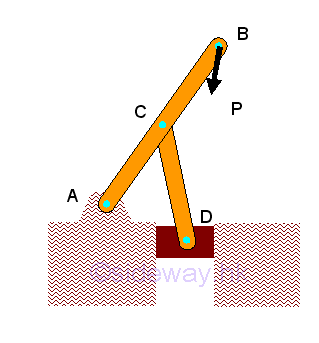Machine is the most common type of mechanical structure designed to transmit and modify forces. In general, machine likes frame structure always contain at least one multiple force member with forces, which are not all along the direction of member axis, acts on  three or more positions the machine member. The main difference between machine and frame is the feature of the component parts. A machine always contain moving component parts. Therefore machine can be considered as a structure in the form of a simple tool or complicated mechanism designed for a specific mechanical function to transform input forces into output forces as per the mechancial function required.

## Stability:

Since moving component parts are always included in machine design, a machine structure must be nonrigid. However, the supports and connections, and external applied force and loading of the machine always provide additional constraints to stabilize the shape of the machine. Therefore machine structure is usually unstable when the external forces applied to the machine structure are not equal to the specific design conditions.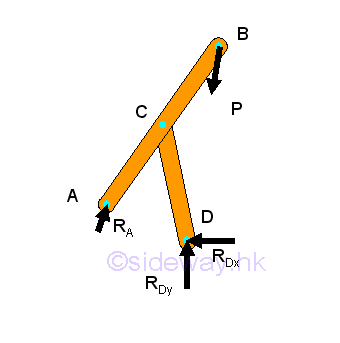## Determinacy:

Since machine is considered as a nonrigid body, the three equilibrium equations of a rigid body usually cannot be used to determine a nonrigid machine. However, a nonrigid machine can usually be divided into rigid component parts. And unknown forces can therefore be determined using the three equilibrium equations of a rigid body by considering each rigid component part as a free body as in collapsible frame structure.

As in collapsible frame, a non-rigid, collapsible structure with suitable constraints is rigid and statically determinate. In general, the necessary condition for a structure to be rigid and statically determinate is the number of unknown forces equal to the number of the independent equations. And the structure should also be properly constrained. The equilibrium equations and unknowns of the machine can be obtained from drawing free-body diagram for each rigid component parts or for each machine member.

If there are more unknown forces than the independent equilibrium equations, the structure is statically indeterminate. If there are more independent equilibrium equations than unknown forces, the structure is unstable, or non-rigid. Only if the number of unknown forces is equal to the number of independent equilibrium equations, the unknown forces can be determined. If all unknowns can be determined and all independent equilibrium equations can be satisfied under general loading conditions, the structure is said to be statically determinate and rigid. However, if there is improper arrangement of frame members and supports, all unknowns cannot be determined and all equilibrium equations cannot be satisfied, and the structure is said to be statically indeterminate and non-rigid.

## Forces Analysis:

Since the machine is collapsible after detached from the supports and connections, the machine cannot be considered as a rigid body. And in general, the three equilibrium equations of a rigid body also cannot determined the four unknown reactions of the collapsible machine.

Forces in machine members can therefore usually be determined by considering the machine structure as distinct component parts. Forces in a two-force member can only be either tensile or compressive forces. Forces acting upon the multi-force member are to be determined. Forces acting on a connecting joint should be in equilibrium.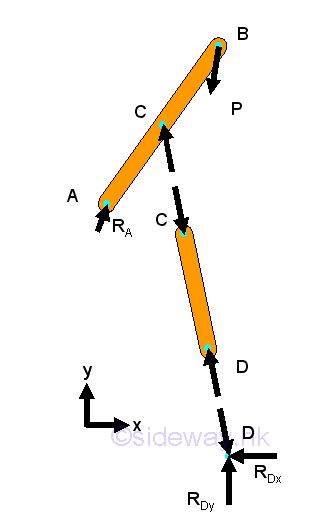At connecting joint D,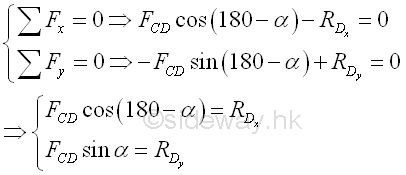For the multiple force machine member AD,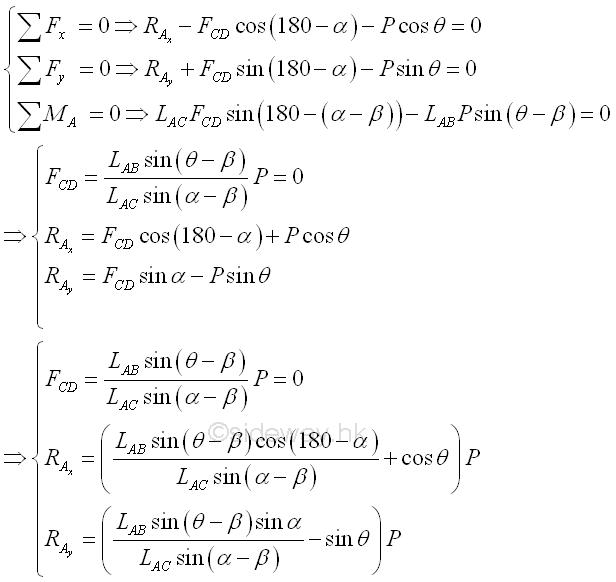©sidewayReferences

1. I.C. Jong; B.G. rogers, 1991, Engineering Mechanics: Statics and Dynamics, Saunders College Publishing, United States of America
2. F.P. Beer; E.R. Johnston,Jr.; E.R. Eisenberg, 2004, Vector Mechanics for Engineers: Statics, McGraw-Hill Companies, Inc., New YorkID: 120300017 Last Updated: 2012/3/27 Revision: 0 Ref:Home (5)

Business

Management

HBR (3)

Information

Recreation

Hobbies (7)

Culture

Chinese (1097)

English (336)

Reference (66)

Computer

Hardware (149)

Software

Application (187)

Digitization (24)

Numeric (19)

Programming

Web (648)CSS (SC)

ASP.NET (SC)

HTML

Knowledge Base

Common Color (SC)

Html 401 Special (SC)

OS (389)

MS Windows

Windows10 (SC)

.NET Framework (SC)

DeskTop (7)

Knowledge

Mathematics

Formulas (8)

Number Theory (206)

Algebra (20)

Trigonometry (18)

Geometry (18)

Calculus (67)

Complex Analysis (21)

Engineering

Tables (8)

Mechanical

Mechanics (1)

Rigid Bodies

Statics (92)

Dynamics (37)

Fluid (5)

Control

Acoustics (19)

Biology (1)

Geography (1)

Copyright © 2000-2019 Sideway . All rights reserved Disclaimers last modified on 10 Feb 2019